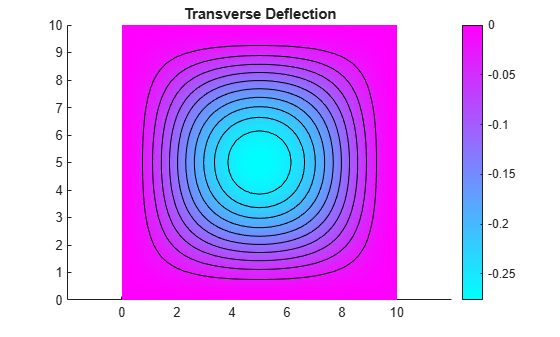Documentation

### This is machine translation

Mouseover text to see original. Click the button below to return to the English version of the page.

To view all translated materials including this page, select Country from the country navigator on the bottom of this page.

## Clamped, Square Isotropic Plate with Uniform Pressure Load

This example shows how to calculate the deflection of a structural plate acted on by a pressure loading.

### PDE and Boundary Conditions For A Thin Plate

The partial differential equation for a thin, isotropic plate with a pressure loading is

``

whereis the bending stiffness of the plate given by

``

andis the modulus of elasticity,is Poisson's ratio, andis the plate thickness. The transverse deflection of the plate isandis the pressure load.

The boundary conditions for the clamped boundaries areandwhereis the derivative ofin a direction normal to the boundary.

The Partial Differential Equation Toolbox™ cannot directly solve the fourth order plate equation shown above but this can be converted to the following two second order partial differential equations.

``

``

whereis a new dependent variable. However, it is not obvious how to specify boundary conditions for this second order system. We cannot directly specify boundary conditions for bothand. Instead, we directly prescribeto be zero and use the following technique to definein such a way to insure thatalso equals zero on the boundary. Stiff "springs" that apply a transverse shear force to the plate edge are distributed along the boundary. The shear force along the boundary due to these springs can be writtenwhereis the normal to the boundary andis the stiffness of the springs. The value ofmust be large enough thatis approximately zero at all points on the boundary but not so large that numerical errors result because the stiffness matrix is ill-conditioned. This expression is a generalized Neumann boundary condition supported by Partial Differential Equation Toolbox™

In the Partial Differential Equation Toolbox™ definition for an elliptic system, theanddependent variables are u(1) and u(2). The two second order partial differential equations can be rewritten as

``

``

which is the form supported by the toolbox. The input corresponding to this formulation is shown in the sections below.

### Create the PDE Model

Create a pde model for a PDE with two dependent variables.

```numberOfPDE = 2; model = createpde(numberOfPDE);```

### Problem Parameters

```E = 1.0e6; % modulus of elasticity nu = .3; % Poisson's ratio thick = .1; % plate thickness len = 10.0; % side length for the square plate hmax = len/20; % mesh size parameter D = E*thick^3/(12*(1 - nu^2)); pres = 2; % external pressure```

### Geometry Creation

For a single square, the geometry and mesh are easily defined as shown below.

```gdm = [3 4 0 len len 0 0 0 len len]'; g = decsg(gdm,'S1',('S1')');```

Create a geometry entity.

`geometryFromEdges(model,g);`

Plot the geometry and display the edge labels for use in the boundary condition definition.

```figure; pdegplot(model,'EdgeLabels','on'); ylim([-1,11]) axis equal title 'Geometry With Edge Labels Displayed';```### Coefficient Definition

The documentation on PDE coefficients shows the required formats for the a and c matrices. The most convenient form for c in this example isfrom the table whereis the number of differential equations. In this example. Thetensor, in the form of anmatrix ofsubmatrices is shown below.

``

The six-row by one-column c matrix is defined below. The entries in the fulla matrix and thef vector follow directly from the definition of the two-equation system shown above.

```c = [1 0 1 D 0 D]'; a = [0 0 1 0]'; f = [0 pres]'; specifyCoefficients(model,'m',0,'d',0,'c',c,'a',a,'f',f);```

### Boundary Conditions

`k = 1e7; % spring stiffness`

Define distributed springs on all four edges.

```bOuter = applyBoundaryCondition(model,'neumann','Edge',(1:4),... 'g',[0 0],'q',[0 0; k 0]);```

### Mesh generation

`generateMesh(model, 'Hmax', hmax);`

### Finite Element and Analytical Solutions

The solution is calculated using the `solvepde` function and the transverse deflection is plotted using the `pdeplot` function. For comparison, the transverse deflection at the plate center is also calculated using an analytical solution to this problem.

```res = solvepde(model); u = res.NodalSolution; numNodes = size(model.Mesh.Nodes,2); figure pdeplot(model,'XYData',u(1:numNodes),'Contour','on'); title 'Transverse Deflection'``````numNodes = size(model.Mesh.Nodes,2); fprintf('Transverse deflection at plate center(PDE Toolbox) = %12.4e\n',... min(u(1:numNodes,1)));```
```Transverse deflection at plate center(PDE Toolbox) = -2.7632e-01 ```

Compute analytical solution.

```wMax = -.0138*pres*len^4/(E*thick^3); fprintf('Transverse deflection at plate center(analytical) = %12.4e\n', wMax);```
```Transverse deflection at plate center(analytical) = -2.7600e-01 ```
##### SupportGet trial now